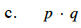Home > CCA2 > Chapter 11 > Lesson 11.2.3 > Problem11-69

11-69.
1. Let p = 2 + 5i and q = 3 − 4i.
Calculate the following values and simplify to a + bi form. Homework Help ✎

1. p + q

2. pq

3. pq

4.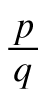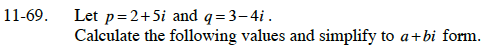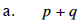Substitute the known values into the equation for p and q.

(2 + 5i) + (3 − 4i)

Combine like terms.

(2 + 3) + (5i − 4i)

Simplify.

5 + i

Is this in a + bi form? How can you tell?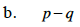Use the same method as part (a).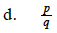−0.56 + 0.92i

${\text{Remember that }i^2 = -1.}$

Use a generic rectangle to multiply the binomials.

26 + 27i